# LIC AAO Mains Reasoning Questions 2019 (Day-11)

Dear Aspirants, Our IBPS Guide team is providing new series of Reasoning Questions for LIC AAO Mains 2019 so the aspirants can practice it on a daily basis. These questions are framed by our skilled experts after understanding your needs thoroughly. Aspirants can practice these new series questions daily to familiarize with the exact exam pattern and make your preparation effective.

[WpProQuiz 6489]

Directions (1-5): Study the given information carefully and answer the following questions.

Certain numbers of people are sitting in a Circular table facing towards the centre of the table. Only few of them names and seating positions are known.

H sits immediate right of S. No known person sits near to U. K is not an immediate neighbour of D. There are seven person sits between H and T when counted from the left of T. Number of person sits between U and H when counted from the right of H is equal to the number of persons sits between I and T when counted from the left of T. T is an immediate neighbour of P. K sits third to the left of A who sits sixth to the right of P. Only one person sits between P and D. Only two people sit between A and H. N sits third to the right of S.

1) What is the position of U with respect to D?

a) Third to the right

b) Second to the left

c) Third to the left

d) Immediate right

e) None of those given as option

2) Which of the following statement is true?

a) N sits second to the right of I

b) T is immediate neighbour of I

c) A sits seventh to the right of D

d) K sits seventh to the left of N

e) None of the statement is correct

3) Number of person sits between U and N when counted from the left of U is same as Number of person sits between H and ______ when counted from right of H?

a) A

b) I

c) D

d) P

e) T

4) How many unknown person sits in the table?

a) 8

b) 10

c) 19

d) 18

e) None of these

5) Who sits second to the right of A?

a) The one who sits immediate right of H

b) The one who sits eighth to the right of P

c) The one who sits fourth to the right of I

d) D

e) None of these

Directions (6 -9): Study the following information to answer the given questions

A number arrangement machine when given an input line of numbers rearranges them following a particular rule. The following is an illustration of input and re-arrangement.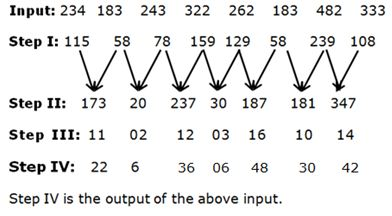As per the rules followed in the above steps, find out in each of the following questions the appropriate steps for the given input.

Input:  105           164   333   584   222    489   176   267

6) Which of the following element is second to the right of the element which is sixth from the right end in step III?

a) 30

b) 10

c) None of these

d) 33

e) 28

7) Which of the following is the Middle element in the last step?

a) 30

b) 66

c) 36

d) 34

e) None of these

8) What will be the resultant if third element from the left end in step II is multiplied by Second element from the right end in step III?

a) 4278

b) 4378

c) 4468

d) None of these

e) 3378

9) If all the numbers in the step IV are arranged in ascending order from left to right, then how many numbers are not changed from its previous position?

a) One

b) Two

c) Three

d) None

e) More than Three

10) The questions below has question and followed by two statements I and II. You have to decide whether the data provided in the statements are sufficient to answer the question. Read the question and both the statements and give answer.

Common data: Six persons A, B, C, D, E and F are lives in six storeys building but not necessarily in the same order. The topmost floor is numbered 1 and bottommost floor is numbered 6. Who lives in third floor?

Statement I: Only two person lives between E and F. Only one person lives between F and A. C lives immediately above E but not above F.

Statement II: F lives in odd number floor. Number of person lives above A is as same as number of person lives below D.

a) The data in statement I alone are sufficient to answer the question, while the data in statement II alone are not sufficient to answer the question.

b) The data in statement II alone are sufficient to answer the question, while the data in statement I alone are not sufficient to answer the question.

c) The data in either in statement I alone or statement II alone are sufficient to answer the question.

d) The data in both statements I and II are not sufficient to answer the question.

e) The data in both statements I and II are sufficient to answer the question.

Directions (1-5):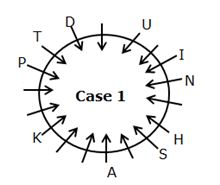K sits third to the left of A, who sits sixth to the right of P. Only one person sits between P and D. K is not an immediate neighbour of D.T is an immediate neighbour of P.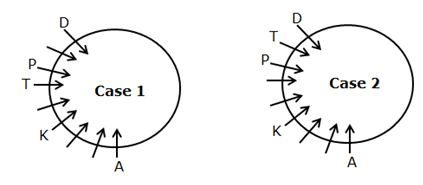There are seven person sits between H and T when counted from the left of T. Only two people sits between A and H. H sits immediate right of S.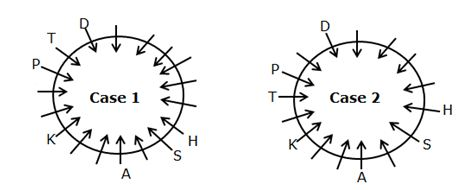Number of person sits between U and H when counted from the right of H is equal to the number of persons sits between I and T when counted from the left of T. No known person sits near to U. N sits third to the right of S.

From this statement we cannot fix the position of U in case 2. So this case is eliminated.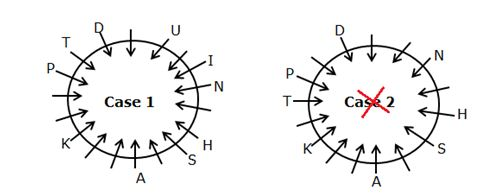Directions (6 -9):

Step I: Even number is divided by 2 and then subtract by 2, Odd number is divided by 3 and then subtract by 3

Step II: +, – , +, -, +, – , +,

Step III: Sum of all the digits of each number

Step IV: Odd Number is multiplied by 2, Even number is multiplied by 3.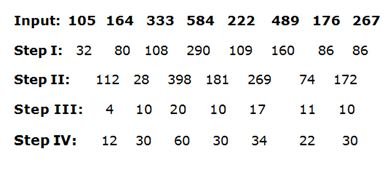From Statement I we get two possibilities,

• There are only two person lives between E and F.
• Only one person lives between F and A.
• C lives immediately above E.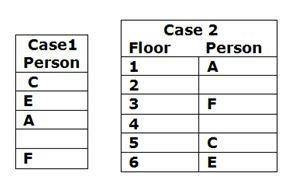From Statement-II,

• F lives in odd number floor.
• Number of person lives above A is as same as number of person lives below D.
• In case 2 we cannot fix the position of D and A.

So, the data in statement I alone are sufficient to answer the question.